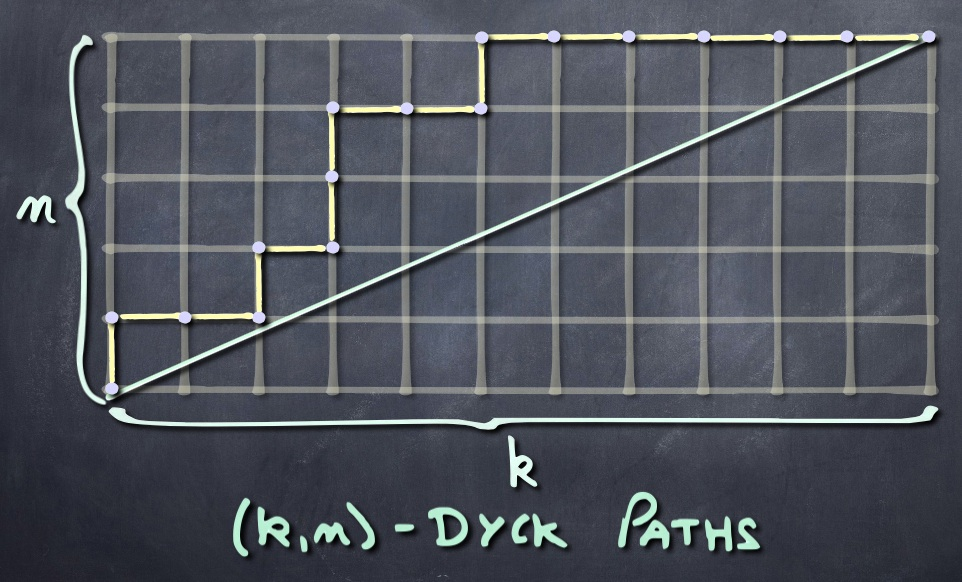# $(q,t)$-Catalan polynomialsThe $q,t$-Catalan polynomials $C_n(q,t)$ lie in $\mathbb{N}[q,t].$ They specialize to the classical Catalan numbers at $q=t=1$. For more on these numbers and their history, see this page. One may also obtain the two classical $q$-analogs of Catalan number by a suitable specialization of $t$. More precisely, at $t=1$ one obtains the $q$-polynomial $C_n(q):=C_n(q,1)$ that satisfies the recurrence

$\displaystyle C_{n+1}(q)=\sum_{k=0}^{n} q^k\,C_k(q)\,C_{n-k}(q),\qquad {\rm with}\qquad C_0(q)=1,$

whereas the other classical $q$-analog may be obtained as

$\displaystyle q^{\binom{n}{2}}\,C_n(q,1/q) = \frac{1}{[n+1]_q} \genfrac{[}{]}{0pt}{}{2\,n}{n}_q$

with the right hand side expressed in the usual $q$-analog notations.

For small values of $n$, one has

$C_{{1}} ( q,t ) =1$
$C_{{2}} ( q,t ) =t+q$
$C_{{3}} ( q,t ) =qt+{t}^{3}+q{t}^{2}+{q}^{2}t+{q}^{3}$
$C_{{4}} ( q,t ) =q{t}^{3}+{q}^{2}{t}^{2}+{q}^{3}t+q{t}^{4}+{q}^{2}{t}^{3}+{q}^{3}{t}^{2}+{q}^{4}t\\ \qquad+{t}^{6}+q{t}^{5}+{q}^{2}{t}^{4}+{q}^{3}{t}^{3}+{q}^{4}{t}^{2}+{q}^{5}t+{q}^{6}$

More values here.

Original definition (an alternate elementary recursive description is given below)

The $q,t$-Catalan polynomials appear as bigraded enumerators for the alternating component of the $\mathbb{S}_n$-module of diagonal harmonic polynomials (see also the $\nabla$ operator). Hence they are defined as

$C_{{n}} ( q,t ):=\langle\nabla(e_n(\mathbf{x}),e_n(\mathbf{x})\rangle,$

where $\langle-,-\rangle$ is the usual scalar product on symmetric functions, and $e_n(\mathbf{x})$ stands for the elementary symmetric function. It follows that they are symmetric in $q$ and $t$, but there is not yet a “direct” combinatorial proof of this fact.

Elementary recursive description (see Garsia-Haglund 2000)

The $C_n(q,t)$ polynomials may be directly calculated as follows. One considers auxiliary polynomials $H_{n,k}(q,t)$, with $k\leq n$, that are recursively defined as

$\displaystyle H_{n,k}(q,t)=t^{n-k}q^{\binom{k}{2}}\sum_{r=1}^{n-k}\genfrac{[}{]}{0pt}{}{r+k-1}{r}_qH_{n-k,r}(q,t),$

with initial condition $H_{n,n}(q,t)=q^{\binom{n}{2}}$. Then we have

$C_n(q,t)=t^{-n}H_{n+1,1}(q,t).$All of these considerations extend to polynomials that specialize to the $(k,n)$-Catalan numbers, $\mathcal{C}_{k,n}$, who enumerate paths that remain above the diagonal in the $(k\times n)$-rectangle. When $k$ and $n$ are coprime, these $(k,n)$-Catalan numbers are simply given by the formula (easily obtained using the Cycle lemma of Dvoretsky and Motzkin)

$\mathcal{C}_{k,n}=\displaystyle\frac{1}{k+n}\binom{k+n}{k}.$

This corresponds to the classical case if one chooses $k=n+1$. More generally, the Fuss-Catalan numbers correspond to $k=mn+1$. When $k$ and $n$ are not coprime, one may use the following  Bizley’s formula (in generating function format) to calculate $\mathcal{C}_{k,n}$. Let $(k,n)=(da,db)$, with $d=\mathrm{gcd}(k,n)$ (hence $a$ and $b$ are coprime), then

$\displaystyle\sum_{d\geq 0}\mathcal{C}_{da,db}\,x^d=\exp\left(\sum_{j\geq 1}\binom{ja+jb}{ja}\frac{x^j}{ja+jb}\right).$

For more on this, see